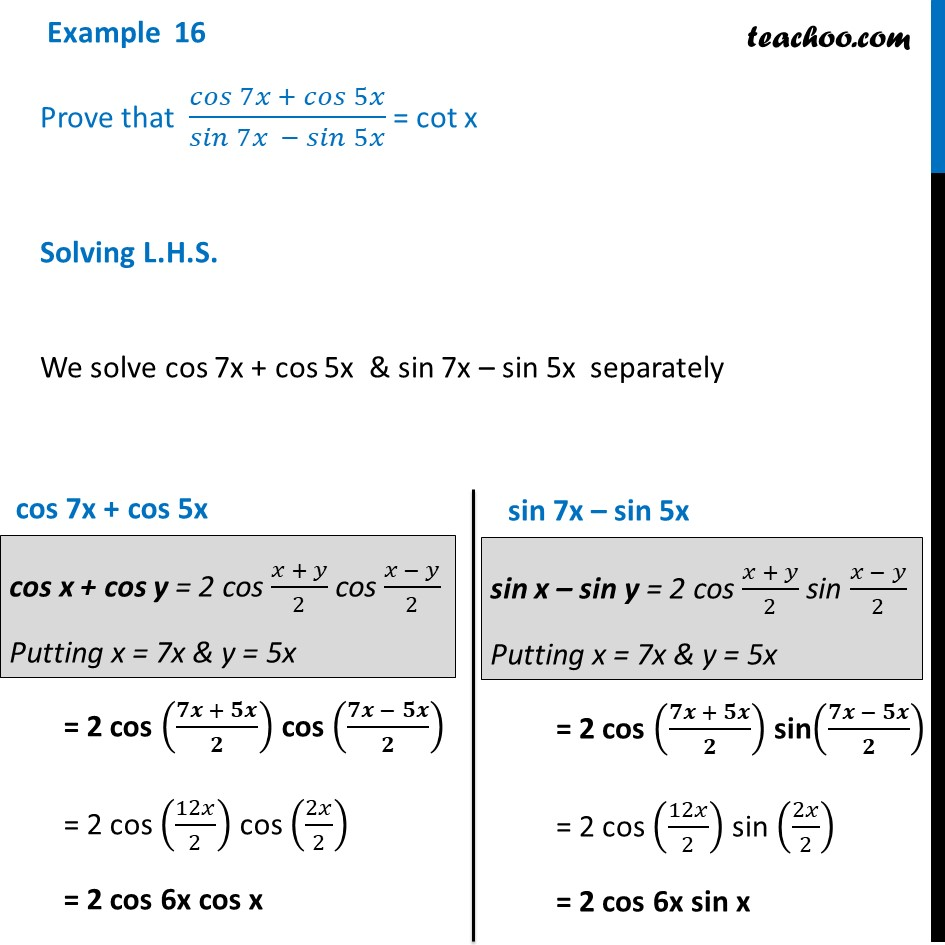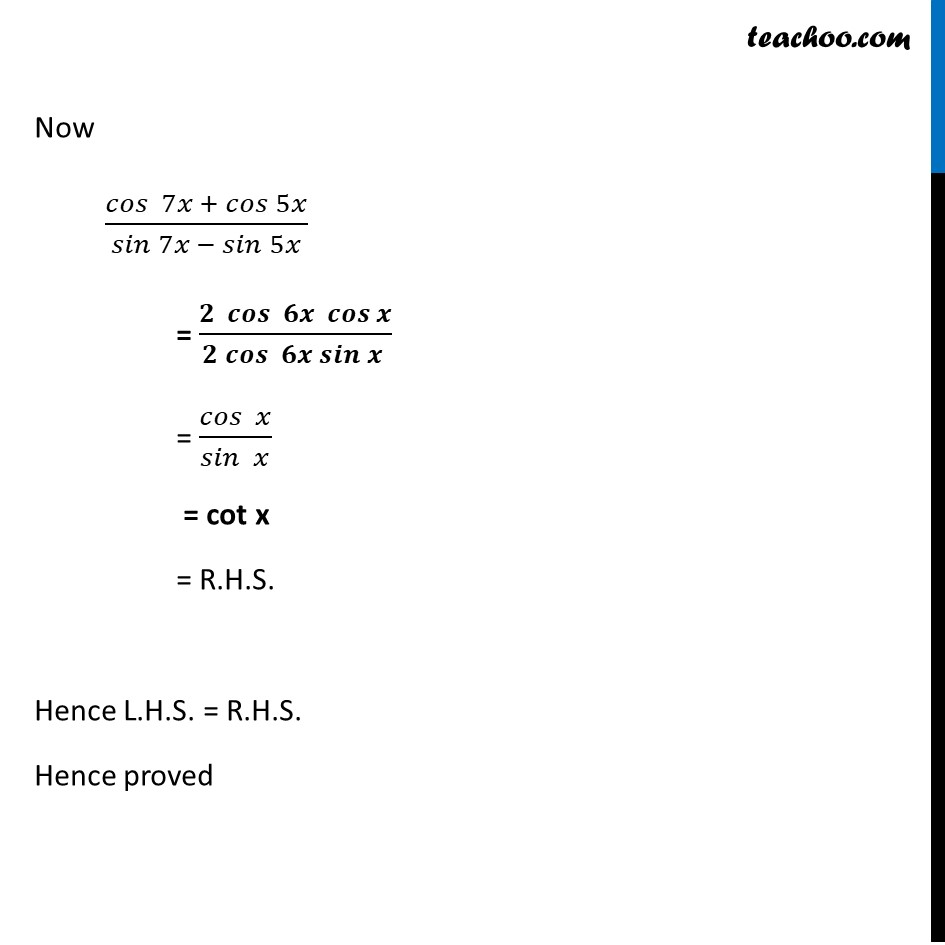Examples

Chapter 3 Class 11 Trigonometric Functions
Serial order wiseLearn in your speed, with individual attention - Teachoo Maths 1-on-1 Class

### Transcript

Example 16 Prove that 𝑐𝑜𝑠⁡〖7𝑥 + 𝑐𝑜𝑠⁡5𝑥 〗/𝑠𝑖𝑛⁡〖7𝑥 − 𝑠𝑖𝑛⁡5𝑥 〗 = cot x Solving L.H.S. We solve cos 7x + cos 5x & sin 7x – sin 5x separately cos 7x + cos 5x = 2 cos ((𝟕𝒙 + 𝟓𝒙)/𝟐) cos ((𝟕𝒙 − 𝟓𝒙)/𝟐) = 2 cos (12𝑥/2) cos (2𝑥/2) = 2 cos 6x cos x sin 7x – sin 5x = 2 cos ((𝟕𝒙 + 𝟓𝒙)/𝟐) sin((𝟕𝒙 − 𝟓𝒙)/𝟐) = 2 cos (12𝑥/2) sin (2𝑥/2) = 2 cos 6x sin x Now 〖𝑐𝑜𝑠 〗⁡〖7𝑥 + 𝑐𝑜𝑠⁡5𝑥 〗/𝑠𝑖𝑛⁡〖7𝑥 − 𝑠𝑖𝑛⁡5𝑥 〗 = (𝟐 〖 𝒄𝒐𝒔 〗⁡〖𝟔𝒙 𝒄𝒐𝒔⁡𝒙 〗)/(𝟐 𝒄𝒐𝒔⁡〖 𝟔𝒙 𝒔𝒊𝒏⁡𝒙 〗 ) = 𝑐𝑜𝑠⁡〖 𝑥〗/𝑠𝑖𝑛⁡〖 𝑥〗 = cot x = R.H.S. Hence L.H.S. = R.H.S. Hence proved